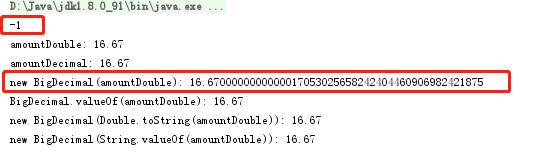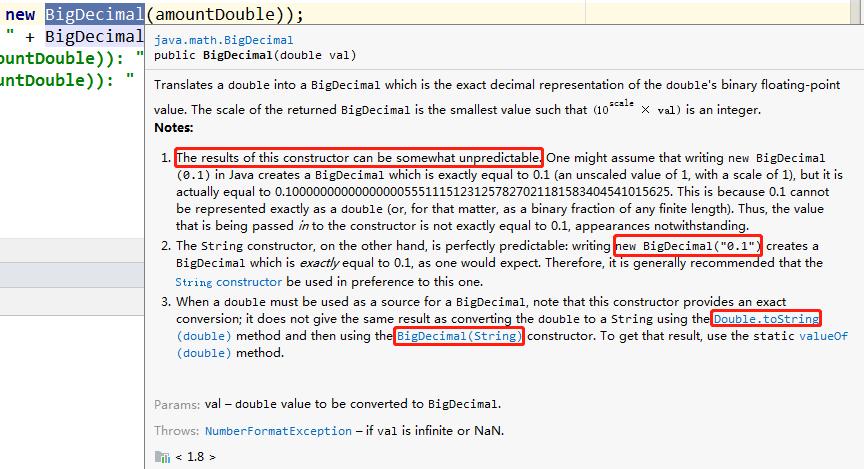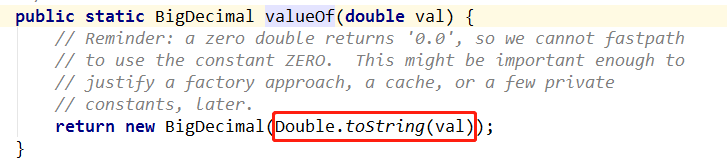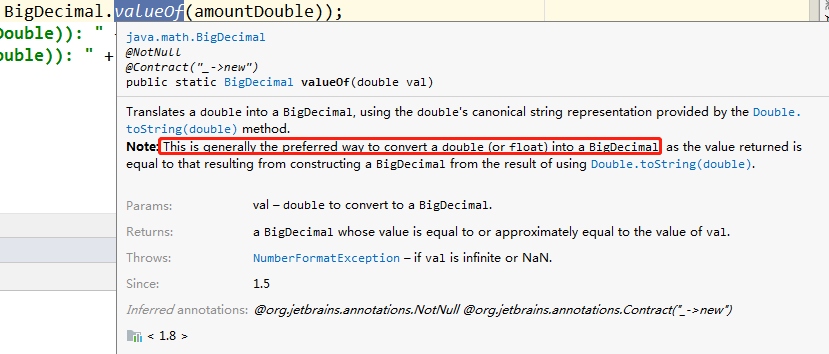# Java中double转BigDecima导致精度损失

## 1.楔子

        @Test
public void doubleToDecimal() {
double amountDouble = 16.67;
BigDecimal amountDecimal = new BigDecimal("16.67");
System.out.println(amountDecimal.compareTo(new BigDecimal(amountDouble)));

System.out.println("amountDouble: " + amountDouble);
System.out.println("amountDecimal: " + amountDecimal);
System.out.println("new BigDecimal(amountDouble): " + new BigDecimal(amountDouble));
System.out.println("BigDecimal.valueOf(amountDouble): " + BigDecimal.valueOf(amountDouble));
System.out.println("new BigDecimal(Double.toString(amountDouble)): " + new BigDecimal(Double.toString(amountDouble)));
System.out.println("new BigDecimal(String.valueOf(amountDouble)): " + new BigDecimal(String.valueOf(amountDouble)));
}## 2.分析和正确使用姿势

float和double类型的主要设计目标是为了科学计算和工程计算。他们执行二进制浮点运算，这是为了在广域数值范围上提供较为精确的快速近似计算而精心设计的。然而，它们没有提供完全精确的结果，所以不应该被用于要求精确结果的场合。正式场合应该使用BigDecimal。

BigDecimal的构造函数public BigDecimal(double val)会损失了double 参数的精度。jdk中已经明确不建议使用new BigDecimal(double value)这种形式的构造函数，而是使用new BigDecimal(Stringvalue)BigDecimal.valueof( double value)

new BigDecimal(double value) javadoc如下(已经说的很明确了，会出现不可预测的情况)，不推介使用：BigDecimal.valueof( double value) 源码如下：## 4.扩展阅读©️2019 CSDN 皮肤主题: 技术黑板 设计师: CSDN官方博客